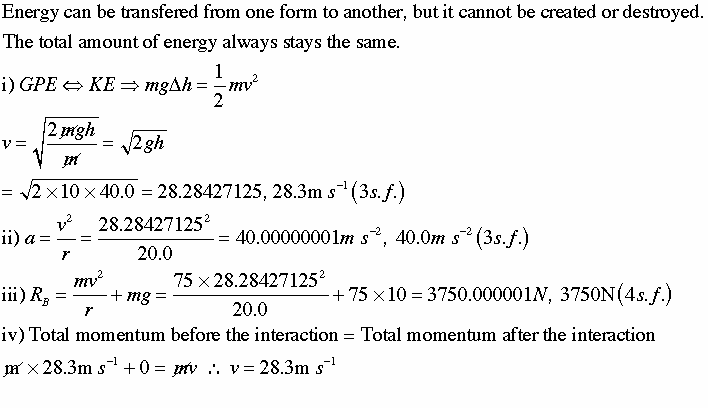# Q10A4-physics-big dipper

Hi:
I have a question to answer but I don't think I have enough information.
I know I have to consider that GPE goes to KE, with max.velocity at the bottom of the dip. I think that the amplitude is the radius of the dip. And I think it is important to think about Total energy is proportional to amplitude squared. But... I'm stuck how to start this without knowing the mass really.
any help appreciated. thank you.

On a fairground big dipper, a train free-wheels down a steep incline into a dip before rising out of the dip. The track at the dip has a radius of curvature of 20.0 m. Assuming the train is almost stationary at the top of the incline before descending, and given that the train drops 20.0m, calculate
(i) the maximum speed the train could have at the dip.
(ii) the centripetal acceleration of the train as it passes through the dip.
(iii) the normal reaction force on a passenger of mass 75kg at this point.
Unfortunately, another train is stationary at the bottom of the dip and a collision occurs in which the two trains move as one up the rise following the dip.
(iv) how far vertically will the combined two-part train go up the rise?

## The Attempt at a Solution

ideasrule
Homework Helper
Do you know the equations for gravitational potential energy and kinetic energy? Can you write out the conservation of energy formula?

Thank you:
I have done some calculations - if you could check please.
The last part of the question is...
Not sure. I started with conservation of momentum and collisions, but actually, I think it doesn't apply here. The question says 'how far vertically'. So again, is it a change back from KE to GPE? And what about any loss of energy in the collision?